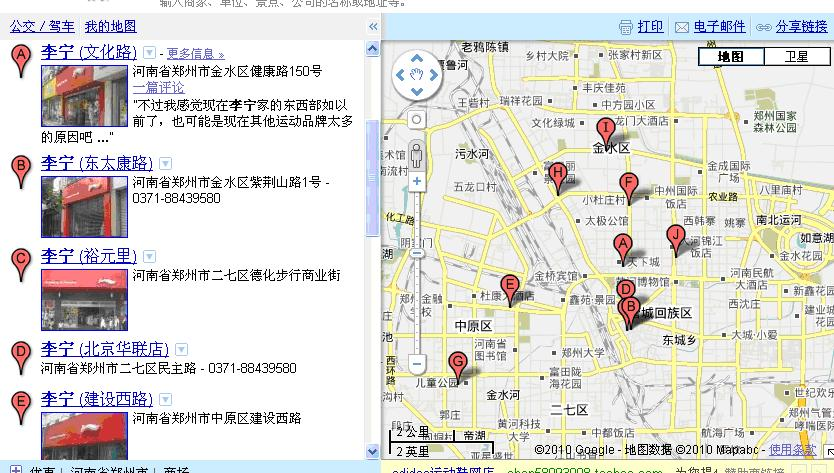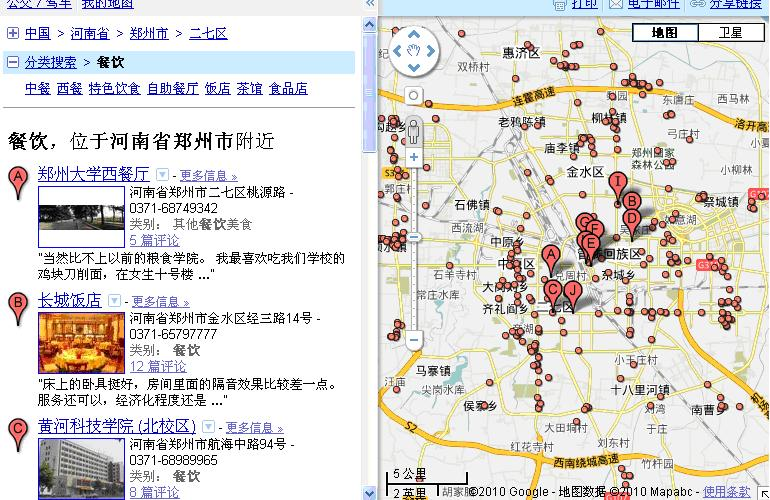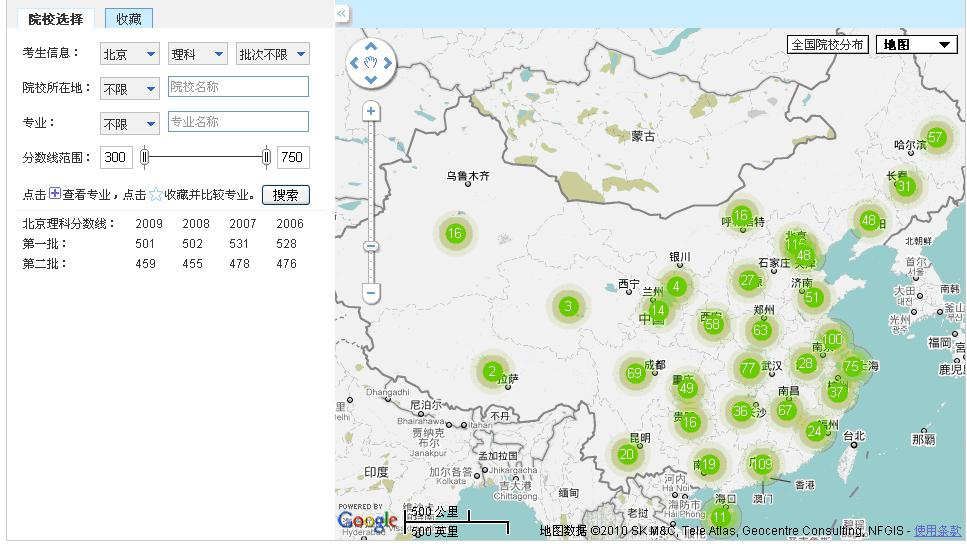1、 减少数据库中读取出来的数据进行。

2、 在前台建立格网索引，加快计算压盖速度。12级为16*16的 格网

13级为32*32的 格网

….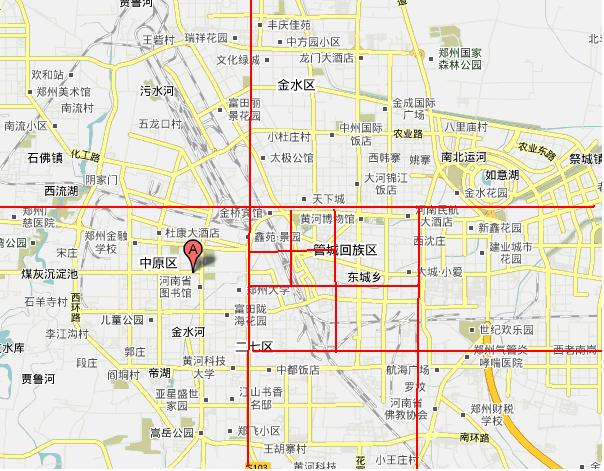字段 类型描述 信息id 关联的信息id Lev12 整型格网号 Lev13 整型格网号 Lev14 整型格网号 Lev15 整型格网号 Lev16 整型格网号 Lev17 整型格网号 Lev18 整型格网号 Lev19 整型格网号

insert into shopindex (shopid) values (sid);

update shopindex s

set (lev12, lev13, lev14, lev15, lev16, lev17, lev18, lev19) = (select GetGirdNo(px,

py,

1212) lev12,

GetGirdNo(px,

py,

1312) lev13,

GetGirdNo(px,

py,

1412) lev14,

GetGirdNo(px,

py,

1512) lev15,

GetGirdNo(px,

py,

1612) lev16,

GetGirdNo(px,

py,

1712) lev17,

GetGirdNo(px,

py,

1812) lev18,

GetGirdNo(px,

py,

1912) lev19

from ooshop f

where s.shopid = f.id)

where s.shopid = sid;

create or replace function GETGIRDNO(x in integer,

y in integer,

lev in integer)

return integer is

girdnum INT := 15;

width INT := 110866;

height INT := 77900;

gnum INT;

stpwidth int;

stpheight int;

xmin int := 40869800;

ymin int := 12467548;

col int;

row int;

res int;

BEGIN

gnum := girdnum * POWER(2, lev);

stpwidth := width / gnum;

stpheight := height / gnum;

col := (x – xmin) / stpwidth;

row := (y – ymin) / stpheight;

res := col + row * gnum;

if res < 0 or res >girdnum*girdnum * POWER(4, lev) then

res := 0;

end if;

return res;

END GetGirdNo;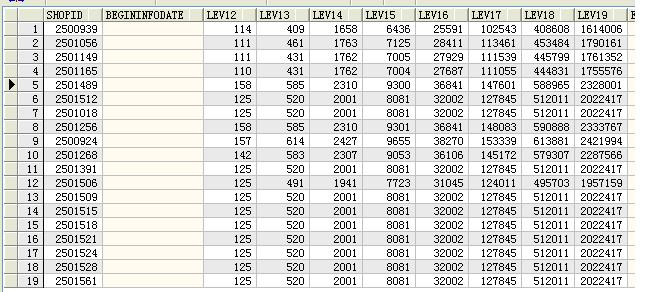select lev12,count(shopid) from shopindex t group by lev12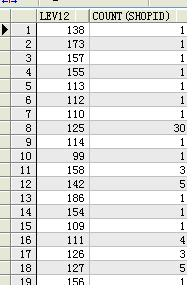create or replace function GETGIRDNOS(stcol in integer,

strow in integer,

encol in integer,

enrow in integer,

lev   in integer)

RETURN colrows IS

gnum    int;

i       int;

j       int;

girdnum INT := 15;

colrow  colrows := colrows();

BEGIN

i := strow;

gnum := girdnum * POWER(2, lev);

while (i <= enrow) loop

j := stcol;

while (j <= encol) loop

colrow.EXTEND;

colrow(colrow.COUNT) := j + i * gnum;

j := j + 1;

end loop;

i := i + 1;

end loop;

RETURN colrow;

END GetGirdNos;

var result = new Collection<ooBFeature>();

{

ooBFeature gm = new ooBFeature(1);

int row = (int)(gm.I / grid.GNum);

int col = (int)(gm.I % grid.GNum);

gm.X = col * grid.StpWidth + grid.StpWidth / 2 + ooGridModel.x;

gm.Y = row * grid.StpHeight + grid.StpHeight / 2 + ooGridModel.y;

}

return result;

### 觉得文章有用就打赏一下文章作者

#### 支付宝扫一扫打赏#### 微信扫一扫打赏• QQ咨询
• 回顶